# Mean State

Period Mean (original grids) [Watt m-2]
Model Period Mean (intersection) [Watt m-2]
Model Period Mean (complement) [Watt m-2]
Benchmark Period Mean (intersection) [Watt m-2]
Benchmark Period Mean (complement) [Watt m-2]
Bias [Watt m-2]
RMSE [Watt m-2]
Phase Shift [months]
Bias Score 
RMSE Score 
Seasonal Cycle Score 
Spatial Distribution Score 
Interannual Variability Score 
Overall Score 
Benchmark [-] -56.8
CRUNCEPv7 [-] -54.2 -54.0 0.00 -57.0 -52.3 3.39 13.3 2.44 0.61 0.46 0.61 0.99 0.54 0.61
GSWP3v1 [-] -64.0 -63.8 0.00 -57.0 -52.3 -7.03 15.1 1.71 0.49 0.46 0.76 0.95 0.82
WFDEI [-] -43.4 -42.9 0.00 -57.0 -52.3 14.0 20.1 2.96 0.35 0.43 0.51 0.97 0.72 0.57
Period Mean (original grids) [Watt m-2]
Model Period Mean (intersection) [Watt m-2]
Model Period Mean (complement) [Watt m-2]
Benchmark Period Mean (intersection) [Watt m-2]
Benchmark Period Mean (complement) [Watt m-2]
Bias [Watt m-2]
RMSE [Watt m-2]
Phase Shift [months]
Bias Score 
RMSE Score 
Seasonal Cycle Score 
Spatial Distribution Score 
Interannual Variability Score 
Overall Score 
Benchmark [-] -87.0
CRUNCEPv7 [-] -84.2 -85.0 0.00 -87.4 -58.4 2.88 17.7 0.342 0.81 0.60 0.97 0.96 0.52 0.74
GSWP3v1 [-] -91.7 -92.4 0.00 -87.4 -58.4 -4.61 18.6 0.227 0.76 0.60 0.98 0.90 0.72
WFDEI [-] -73.9 -74.8 0.00 -87.4 -58.4 12.8 22.0 0.651 0.66 0.58 0.92 1.0 0.72 0.74
Period Mean (original grids) [Watt m-2]
Model Period Mean (intersection) [Watt m-2]
Model Period Mean (complement) [Watt m-2]
Benchmark Period Mean (intersection) [Watt m-2]
Benchmark Period Mean (complement) [Watt m-2]
Bias [Watt m-2]
RMSE [Watt m-2]
Phase Shift [months]
Bias Score 
RMSE Score 
Seasonal Cycle Score 
Spatial Distribution Score 
Interannual Variability Score 
Overall Score 
Benchmark [-] -81.4
CRUNCEPv7 [-] -77.5 -77.7 0.00 -82.3 -62.6 6.42 19.0 1.28 0.68 0.50 0.83 0.98 0.48 0.66
GSWP3v1 [-] -86.5 -86.6 0.00 -82.3 -62.6 -2.65 16.8 0.707 0.71 0.53 0.92 0.96 0.71
WFDEI [-] -76.7 -76.9 0.00 -82.3 -62.6 6.70 19.4 1.14 0.66 0.49 0.85 1.0 0.62 0.68
Period Mean (original grids) [Watt m-2]
Model Period Mean (intersection) [Watt m-2]
Model Period Mean (complement) [Watt m-2]
Benchmark Period Mean (intersection) [Watt m-2]
Benchmark Period Mean (complement) [Watt m-2]
Bias [Watt m-2]
RMSE [Watt m-2]
Phase Shift [months]
Bias Score 
RMSE Score 
Seasonal Cycle Score 
Spatial Distribution Score 
Interannual Variability Score 
Overall Score 
Benchmark [-] -37.2
CRUNCEPv7 [-] -59.2 -59.2 0.00 -36.9 -41.0 -22.2 24.9 1.05 0.26 0.51 0.88 0.79 0.51 0.58
GSWP3v1 [-] -39.1 -39.1 0.00 -36.9 -41.0 -2.99 15.8 1.18 0.73 0.41 0.85 0.97 0.88
WFDEI [-] -55.0 -55.1 0.00 -36.9 -41.0 -18.4 21.5 1.14 0.33 0.51 0.86 0.75 0.53 0.58
Period Mean (original grids) [Watt m-2]
Model Period Mean (intersection) [Watt m-2]
Model Period Mean (complement) [Watt m-2]
Benchmark Period Mean (intersection) [Watt m-2]
Benchmark Period Mean (complement) [Watt m-2]
Bias [Watt m-2]
RMSE [Watt m-2]
Phase Shift [months]
Bias Score 
RMSE Score 
Seasonal Cycle Score 
Spatial Distribution Score 
Interannual Variability Score 
Overall Score 
Benchmark [-] -44.6
CRUNCEPv7 [-] -27.8 -27.7 0.00 -43.2 -49.2 16.1 18.5 3.17 0.096 0.36 0.46 0.84 0.51 0.44
GSWP3v1 [-] -47.9 -47.8 0.00 -43.2 -49.2 -4.20 9.59 1.92 0.38 0.35 0.70 0.98 0.78
WFDEI [-] -21.3 -21.2 0.00 -43.2 -49.2 21.5 22.9 2.56 0.057 0.29 0.59 0.90 0.81 0.49
Period Mean (original grids) [Watt m-2]
Model Period Mean (intersection) [Watt m-2]
Model Period Mean (complement) [Watt m-2]
Benchmark Period Mean (intersection) [Watt m-2]
Benchmark Period Mean (complement) [Watt m-2]
Bias [Watt m-2]
RMSE [Watt m-2]
Phase Shift [months]
Bias Score 
RMSE Score 
Seasonal Cycle Score 
Spatial Distribution Score 
Interannual Variability Score 
Overall Score 
Benchmark [-] -42.1
CRUNCEPv7 [-] -65.9 -65.9 0.00 -41.9 -51.7 -23.9 26.9 0.974 0.23 0.47 0.89 0.74 0.53 0.56
GSWP3v1 [-] -51.6 -51.6 0.00 -41.9 -51.7 -9.58 15.4 0.649 0.56 0.49 0.92 0.98 0.89
WFDEI [-] -62.8 -62.8 0.00 -41.9 -51.7 -20.9 24.5 0.925 0.28 0.46 0.90 0.69 0.53 0.55
Period Mean (original grids) [Watt m-2]
Model Period Mean (intersection) [Watt m-2]
Model Period Mean (complement) [Watt m-2]
Benchmark Period Mean (intersection) [Watt m-2]
Benchmark Period Mean (complement) [Watt m-2]
Bias [Watt m-2]
RMSE [Watt m-2]
Phase Shift [months]
Bias Score 
RMSE Score 
Seasonal Cycle Score 
Spatial Distribution Score 
Interannual Variability Score 
Overall Score 
Benchmark [-] -67.1
CRUNCEPv7 [-] -82.5 -82.3 0.00 -67.3 -59.4 -14.0 22.3 1.24 0.48 0.47 0.83 0.94 0.52 0.62
GSWP3v1 [-] -73.4 -73.1 0.00 -67.3 -59.4 -5.00 16.4 0.937 0.67 0.50 0.87 1.0 0.80
WFDEI [-] -79.1 -79.0 0.00 -67.3 -59.4 -10.8 21.2 1.29 0.55 0.43 0.82 0.98 0.55 0.63
Period Mean (original grids) [Watt m-2]
Model Period Mean (intersection) [Watt m-2]
Model Period Mean (complement) [Watt m-2]
Benchmark Period Mean (intersection) [Watt m-2]
Benchmark Period Mean (complement) [Watt m-2]
Bias [Watt m-2]
RMSE [Watt m-2]
Phase Shift [months]
Bias Score 
RMSE Score 
Seasonal Cycle Score 
Spatial Distribution Score 
Interannual Variability Score 
Overall Score 
Benchmark [-] -71.2
CRUNCEPv7 [-] -68.9 -68.9 0.00 -72.6 -53.4 7.97 20.9 1.14 0.62 0.52 0.86 0.99 0.48 0.66
GSWP3v1 [-] -77.5 -77.7 0.00 -72.6 -53.4 -2.50 17.1 0.848 0.71 0.56 0.89 0.96 0.70
WFDEI [-] -61.0 -61.0 0.00 -72.6 -53.4 14.9 24.5 1.24 0.49 0.50 0.83 0.99 0.63 0.66
Period Mean (original grids) [Watt m-2]
Model Period Mean (intersection) [Watt m-2]
Model Period Mean (complement) [Watt m-2]
Benchmark Period Mean (intersection) [Watt m-2]
Benchmark Period Mean (complement) [Watt m-2]
Bias [Watt m-2]
RMSE [Watt m-2]
Phase Shift [months]
Bias Score 
RMSE Score 
Seasonal Cycle Score 
Spatial Distribution Score 
Interannual Variability Score 
Overall Score 
Benchmark [-] -31.1
CRUNCEPv7 [-] -56.5 -56.6 0.00 -31.1 -32.1 -25.4 27.3 0.813 0.20 0.53 0.93 0.94 0.53 0.61
GSWP3v1 [-] -38.2 -38.3 0.00 -31.1 -32.1 -7.23 15.2 0.805 0.63 0.45 0.92 0.95 0.90
WFDEI [-] -54.1 -54.2 0.00 -31.1 -32.1 -23.1 25.6 1.19 0.24 0.49 0.86 0.89 0.55 0.59
Period Mean (original grids) [Watt m-2]
Model Period Mean (intersection) [Watt m-2]
Model Period Mean (complement) [Watt m-2]
Benchmark Period Mean (intersection) [Watt m-2]
Benchmark Period Mean (complement) [Watt m-2]
Bias [Watt m-2]
RMSE [Watt m-2]
Phase Shift [months]
Bias Score 
RMSE Score 
Seasonal Cycle Score 
Spatial Distribution Score 
Interannual Variability Score 
Overall Score 
Benchmark [-] -96.8
CRUNCEPv7 [-] -93.0 -93.0 0.00 -99.4 -63.9 9.20 20.9 0.539 0.56 0.51 0.92 0.84 0.48 0.64
GSWP3v1 [-] -96.0 -96.1 0.00 -99.4 -63.9 6.20 16.5 0.483 0.67 0.54 0.94 0.92 0.73
WFDEI [-] -92.3 -92.4 0.00 -99.4 -63.9 9.55 22.1 0.465 0.56 0.46 0.94 0.84 0.65 0.65
Period Mean (original grids) [Watt m-2]
Model Period Mean (intersection) [Watt m-2]
Model Period Mean (complement) [Watt m-2]
Benchmark Period Mean (intersection) [Watt m-2]
Benchmark Period Mean (complement) [Watt m-2]
Bias [Watt m-2]
RMSE [Watt m-2]
Phase Shift [months]
Bias Score 
RMSE Score 
Seasonal Cycle Score 
Spatial Distribution Score 
Interannual Variability Score 
Overall Score 
Benchmark [-] -55.6
CRUNCEPv7 [-] -70.5 -70.5 0.00 -55.1 -60.8 -14.9 19.6 0.976 0.34 0.47 0.88 0.53 0.52 0.53
GSWP3v1 [-] -57.8 -57.8 0.00 -55.1 -60.8 -3.30 11.5 0.743 0.66 0.47 0.91 0.96 0.87
WFDEI [-] -66.3 -66.3 0.00 -55.1 -60.8 -11.5 17.4 0.834 0.41 0.44 0.90 0.61 0.55 0.56
Period Mean (original grids) [Watt m-2]
Model Period Mean (intersection) [Watt m-2]
Model Period Mean (complement) [Watt m-2]
Benchmark Period Mean (intersection) [Watt m-2]
Benchmark Period Mean (complement) [Watt m-2]
Bias [Watt m-2]
RMSE [Watt m-2]
Phase Shift [months]
Bias Score 
RMSE Score 
Seasonal Cycle Score 
Spatial Distribution Score 
Interannual Variability Score 
Overall Score 
Benchmark [-] -52.6
CRUNCEPv7 [-] -70.6 -70.6 0.00 -65.2 -46.5 -3.71 22.1 1.25 0.45 0.48 0.83 0.90 0.49 0.60
GSWP3v1 [-] -70.0 -70.0 0.00 -65.2 -46.5 -3.58 16.7 0.949 0.59 0.50 0.87 0.93 0.77
WFDEI [-] -68.2 -68.2 0.00 -65.2 -46.5 -1.70 22.4 1.26 0.44 0.46 0.82 0.90 0.59 0.61
Period Mean (original grids) [Watt m-2]
Model Period Mean (intersection) [Watt m-2]
Model Period Mean (complement) [Watt m-2]
Benchmark Period Mean (intersection) [Watt m-2]
Benchmark Period Mean (complement) [Watt m-2]
Bias [Watt m-2]
RMSE [Watt m-2]
Phase Shift [months]
Bias Score 
RMSE Score 
Seasonal Cycle Score 
Spatial Distribution Score 
Interannual Variability Score 
Overall Score 
Benchmark [-] -121.
CRUNCEPv7 [-] -104. -104. 0.00 -122. -81.3 20.0 32.1 0.849 0.50 0.47 0.89 0.80 0.42 0.59
GSWP3v1 [-] -108. -108. 0.00 -122. -81.3 15.3 25.8 0.675 0.56 0.53 0.91 0.82 0.63
WFDEI [-] -107. -107. 0.00 -122. -81.3 16.2 29.3 0.839 0.55 0.47 0.89 0.75 0.49 0.60
Period Mean (original grids) [Watt m-2]
Model Period Mean (intersection) [Watt m-2]
Model Period Mean (complement) [Watt m-2]
Benchmark Period Mean (intersection) [Watt m-2]
Benchmark Period Mean (complement) [Watt m-2]
Bias [Watt m-2]
RMSE [Watt m-2]
Phase Shift [months]
Bias Score 
RMSE Score 
Seasonal Cycle Score 
Spatial Distribution Score 
Interannual Variability Score 
Overall Score 
Benchmark [-] -34.3
CRUNCEPv7 [-] -60.2 -60.2 0.00 -34.2 -36.5 -25.5 27.3 0.658 0.19 0.52 0.93 0.95 0.50 0.60
GSWP3v1 [-] -42.3 -42.3 0.00 -34.2 -36.5 -8.62 15.2 0.830 0.58 0.46 0.89 0.82 0.88
WFDEI [-] -57.5 -57.5 0.00 -34.2 -36.5 -23.1 25.4 0.649 0.22 0.50 0.93 0.99 0.53 0.61
Period Mean (original grids) [Watt m-2]
Model Period Mean (intersection) [Watt m-2]
Model Period Mean (complement) [Watt m-2]
Benchmark Period Mean (intersection) [Watt m-2]
Benchmark Period Mean (complement) [Watt m-2]
Bias [Watt m-2]
RMSE [Watt m-2]
Phase Shift [months]
Bias Score 
RMSE Score 
Seasonal Cycle Score 
Spatial Distribution Score 
Interannual Variability Score 
Overall Score 
Benchmark [-] -70.7
CRUNCEPv7 [-] -69.5 -69.4 0.00 -73.4 -57.5 9.50 17.8 1.36 0.49 0.48 0.79 0.98 0.51 0.62
GSWP3v1 [-] -81.0 -80.9 0.00 -73.4 -57.5 -4.62 14.4 0.925 0.58 0.49 0.86 0.99 0.79
WFDEI [-] -68.7 -68.7 0.00 -73.4 -57.5 9.08 19.8 1.63 0.47 0.44 0.74 0.95 0.70 0.62
Period Mean (original grids) [Watt m-2]
Model Period Mean (intersection) [Watt m-2]
Model Period Mean (complement) [Watt m-2]
Benchmark Period Mean (intersection) [Watt m-2]
Benchmark Period Mean (complement) [Watt m-2]
Bias [Watt m-2]
RMSE [Watt m-2]
Phase Shift [months]
Bias Score 
RMSE Score 
Seasonal Cycle Score 
Spatial Distribution Score 
Interannual Variability Score 
Overall Score 
Benchmark [-] -53.2
CRUNCEPv7 [-] -66.3 -66.2 0.00 -52.9 -58.5 -12.3 21.3 3.99 0.38 0.29 0.33 0.94 0.62 0.47
GSWP3v1 [-] -60.7 -60.5 0.00 -52.9 -58.5 -7.65 12.0 0.781 0.53 0.48 0.88 1.0 0.89
WFDEI [-] -65.0 -64.8 0.00 -52.9 -58.5 -11.3 19.6 3.21 0.42 0.30 0.47 1.0 0.78 0.54
Period Mean (original grids) [Watt m-2]
Model Period Mean (intersection) [Watt m-2]
Model Period Mean (complement) [Watt m-2]
Benchmark Period Mean (intersection) [Watt m-2]
Benchmark Period Mean (complement) [Watt m-2]
Bias [Watt m-2]
RMSE [Watt m-2]
Phase Shift [months]
Bias Score 
RMSE Score 
Seasonal Cycle Score 
Spatial Distribution Score 
Interannual Variability Score 
Overall Score 
Benchmark [-] -42.5
CRUNCEPv7 [-] -69.0 -69.0 0.00 -42.4 -45.4 -26.5 29.2 0.853 0.21 0.49 0.89 0.73 0.52 0.55
GSWP3v1 [-] -53.7 -53.7 0.00 -42.4 -45.4 -11.0 15.8 0.545 0.53 0.51 0.94 0.99 0.86
WFDEI [-] -66.6 -66.6 0.00 -42.4 -45.4 -24.2 27.1 0.683 0.24 0.49 0.92 0.79 0.56 0.58
Period Mean (original grids) [Watt m-2]
Model Period Mean (intersection) [Watt m-2]
Model Period Mean (complement) [Watt m-2]
Benchmark Period Mean (intersection) [Watt m-2]
Benchmark Period Mean (complement) [Watt m-2]
Bias [Watt m-2]
RMSE [Watt m-2]
Phase Shift [months]
Bias Score 
RMSE Score 
Seasonal Cycle Score 
Spatial Distribution Score 
Interannual Variability Score 
Overall Score 
Benchmark [-] -80.3
CRUNCEPv7 [-] -89.5 -89.2 0.00 -80.8 -54.9 -8.00 21.9 0.511 0.63 0.52 0.94 0.66 0.50 0.63
GSWP3v1 [-] -85.7 -85.5 0.00 -80.8 -54.9 -4.42 18.0 0.271 0.73 0.56 0.97 0.93 0.76
WFDEI [-] -89.1 -88.7 0.00 -80.8 -54.9 -7.63 21.7 0.723 0.66 0.50 0.90 0.77 0.59 0.65
Period Mean (original grids) [Watt m-2]
Model Period Mean (intersection) [Watt m-2]
Model Period Mean (complement) [Watt m-2]
Benchmark Period Mean (intersection) [Watt m-2]
Benchmark Period Mean (complement) [Watt m-2]
Bias [Watt m-2]
RMSE [Watt m-2]
Phase Shift [months]
Bias Score 
RMSE Score 
Seasonal Cycle Score 
Spatial Distribution Score 
Interannual Variability Score 
Overall Score 
Benchmark [-] -43.7
CRUNCEPv7 [-] -31.5 -31.5 0.00 -43.6 -47.7 12.1 16.5 1.66 0.33 0.45 0.77 0.74 0.54 0.54
GSWP3v1 [-] -50.9 -50.8 0.00 -43.6 -47.7 -7.23 12.5 1.59 0.50 0.46 0.78 0.92 0.75
WFDEI [-] -24.9 -24.9 0.00 -43.6 -47.7 18.6 22.0 1.30 0.21 0.41 0.84 0.69 0.76 0.55
Period Mean (original grids) [Watt m-2]
Model Period Mean (intersection) [Watt m-2]
Model Period Mean (complement) [Watt m-2]
Benchmark Period Mean (intersection) [Watt m-2]
Benchmark Period Mean (complement) [Watt m-2]
Bias [Watt m-2]
RMSE [Watt m-2]
Phase Shift [months]
Bias Score 
RMSE Score 
Seasonal Cycle Score 
Spatial Distribution Score 
Interannual Variability Score 
Overall Score 
Benchmark [-] -64.5
CRUNCEPv7 [-] -61.3 -61.7 0.00 -64.8 -56.3 3.81 14.7 1.45 0.62 0.48 0.79 0.77 0.51 0.61
GSWP3v1 [-] -69.7 -70.1 0.00 -64.8 -56.3 -4.85 13.0 0.736 0.71 0.48 0.91 0.97 0.81
WFDEI [-] -57.6 -57.9 0.00 -64.8 -56.3 7.30 16.3 1.35 0.61 0.44 0.81 0.86 0.72 0.65
Period Mean (original grids) [Watt m-2]
Model Period Mean (intersection) [Watt m-2]
Model Period Mean (complement) [Watt m-2]
Benchmark Period Mean (intersection) [Watt m-2]
Benchmark Period Mean (complement) [Watt m-2]
Bias [Watt m-2]
RMSE [Watt m-2]
Phase Shift [months]
Bias Score 
RMSE Score 
Seasonal Cycle Score 
Spatial Distribution Score 
Interannual Variability Score 
Overall Score 
Benchmark [-] -80.0
CRUNCEPv7 [-] -94.0 -93.8 0.00 -83.0 -54.0 -8.53 24.7 1.09 0.42 0.45 0.85 0.61 0.47 0.54
GSWP3v1 [-] -86.3 -85.9 0.00 -83.0 -54.0 -0.276 20.0 0.784 0.53 0.44 0.89 0.89 0.77
WFDEI [-] -91.4 -91.4 0.00 -83.0 -54.0 -6.63 23.1 1.05 0.44 0.43 0.86 0.86 0.54 0.60

# Temporally integrated period mean

BENCHMARK MEAN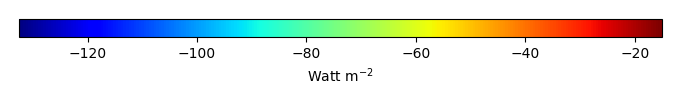MODEL MEANBIAS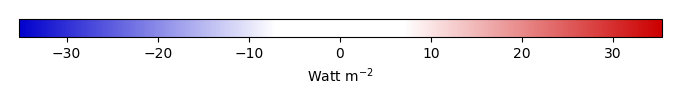BIAS SCORERMSE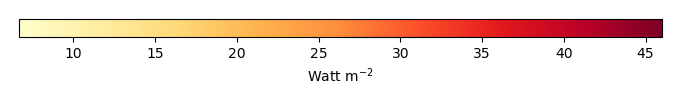RMSE SCOREBENCHMARK INTERANNUAL VARIABILITY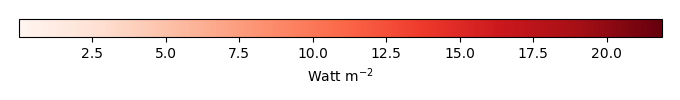MODEL INTERANNUAL VARIABILITYINTERANNUAL VARIABILITY SCOREBENCHMARK MAX MONTHMODEL MAX MONTHDIFFERENCE IN MAX MONTH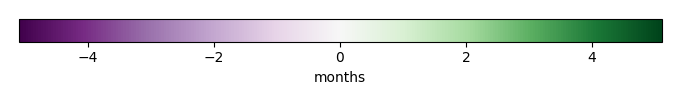SEASONAL CYCLE SCORESPATIAL TAYLOR DIAGRAMMODEL COLORS# Spatially integrated regional mean

MODEL COLORSREGIONAL MEANANNUAL CYCLEMONTHLY ANOMALYANNUAL CYCLE# All Models

BenchmarkCRUNCEPv7GSWP3v1WFDEI# Data Information

creation_date: Tue Jul 1 08:25:00 PDT 2014

source_file: This product is generated from monthly 1 degree GEWEX SRB Radiation observations